### Home > CALC > Chapter Ch3 > Lesson 3.1.2 > Problem3-33

3-33.
1. Expand and evaluate the following sums. Homework Help ✎

1.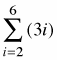2.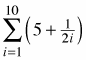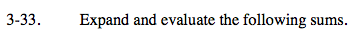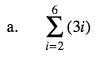=3( 2+3+4+5+6)=3(20)=60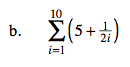How can this sum be rewritten to save you time?